Test: Network Topology

# Test: Network Topology

Test Description

## 10 Questions MCQ Test Topicwise Question Bank for Electrical Engineering | Test: Network Topology

Test: Network Topology for Electrical Engineering (EE) 2023 is part of Topicwise Question Bank for Electrical Engineering preparation. The Test: Network Topology questions and answers have been prepared according to the Electrical Engineering (EE) exam syllabus.The Test: Network Topology MCQs are made for Electrical Engineering (EE) 2023 Exam. Find important definitions, questions, notes, meanings, examples, exercises, MCQs and online tests for Test: Network Topology below.
Solutions of Test: Network Topology questions in English are available as part of our Topicwise Question Bank for Electrical Engineering for Electrical Engineering (EE) & Test: Network Topology solutions in Hindi for Topicwise Question Bank for Electrical Engineering course. Download more important topics, notes, lectures and mock test series for Electrical Engineering (EE) Exam by signing up for free. Attempt Test: Network Topology | 10 questions in 30 minutes | Mock test for Electrical Engineering (EE) preparation | Free important questions MCQ to study Topicwise Question Bank for Electrical Engineering for Electrical Engineering (EE) Exam | Download free PDF with solutions
 1 Crore+ students have signed up on EduRev. Have you?
Test: Network Topology - Question 1

### A possible tree of the topology equivalent of the network shown below is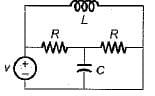Detailed Solution for Test: Network Topology - Question 1

Given network has 4 number of nodes and 5 number of branches.
Hence, it’s tree will be as shown below.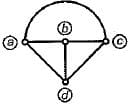Test: Network Topology - Question 2

### In the incidence matrix of a graph

Test: Network Topology - Question 3

### The number of twigs and links in the graph obtained for the given network will be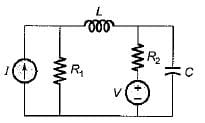Detailed Solution for Test: Network Topology - Question 3

For the given network, number of nodes, n = 3 and number of branches = 4 = b.
Hence, twig s = n - 1 = 3 - 1 = 2 and links = b - { n - 1) = 4 - ( 3 - 1 ) = 2

Test: Network Topology - Question 4

Consider the following statements concerned with the incidence matrix of a graph:
1. Determinant of the incident matrix of a closed loop is non-zero.
2. Algebraic sum of the row entires of an incidence matrix is zero.
3. If branch j in the graph is not incident at node aij = 0,

Which of the above statements is/are true?

Test: Network Topology - Question 5

The number of edges in a complete graph of n-vertices is

Detailed Solution for Test: Network Topology - Question 5

Number of edge =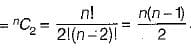Test: Network Topology - Question 6

The number of-branches and nodes in the graph are respectively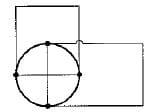Detailed Solution for Test: Network Topology - Question 6

n - 5 and b = 10

Test: Network Topology - Question 7

Assertion (A): When all the elements (i.e. resistors, inductors, capacitors etc.) in a network are replaced by lines with circles or dots at both ends, the configuration is then called the graph of the network.

Reason (R): The graph of any network is drawn by keeping all the points of intersection of two or more branches and representing the network elements of lines, voltages and current sources by their internal impedances.

Test: Network Topology - Question 8

The graph of a network has N number of nodes and B is the number of branches in it.
Match the List -I and List-II and select the correct answer using the codes given below the lists: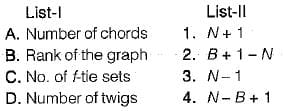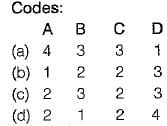Test: Network Topology - Question 9

Two graphs are said to be isomorphic graph if their incidence matrices

Test: Network Topology - Question 10

Assertion (A): The analysis of network is done using Kirchhoffs laws when the values of each element of the network remains constant for all the values of time.

Reason (R): The analysis of a network is done using graph theory when the values of each element of the network are changed with time.

Detailed Solution for Test: Network Topology - Question 10

Assertion is true because if the values of each element is changed w.r.t. time, then every time a fresh KVL and KCL equations are to be written for analysis of the network.
Reason is also true but, the correct explanation of assertion is as explained above, Hence, Reason (R) is not the correct explanation of (A).

## Topicwise Question Bank for Electrical Engineering

207 tests
Information about Test: Network Topology Page
In this test you can find the Exam questions for Test: Network Topology solved & explained in the simplest way possible. Besides giving Questions and answers for Test: Network Topology, EduRev gives you an ample number of Online tests for practice

## Topicwise Question Bank for Electrical Engineering

207 tests(Scan QR code)